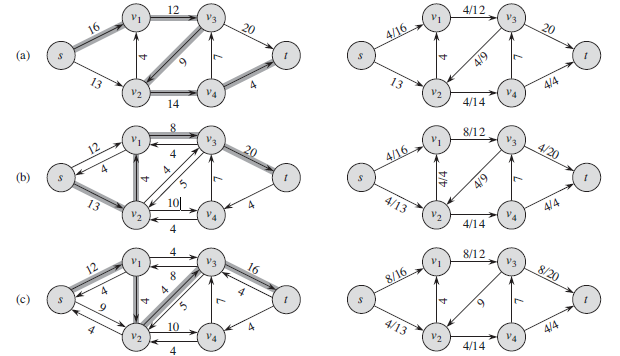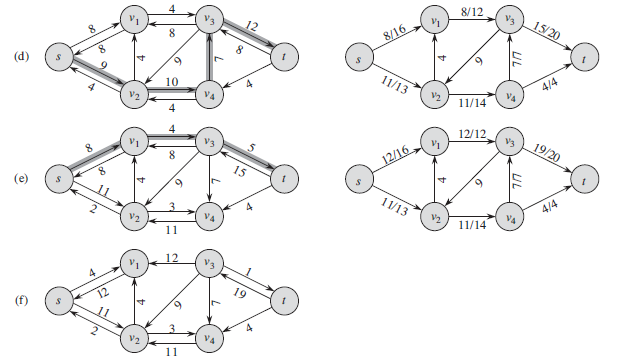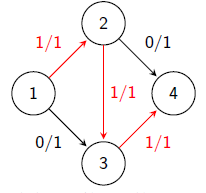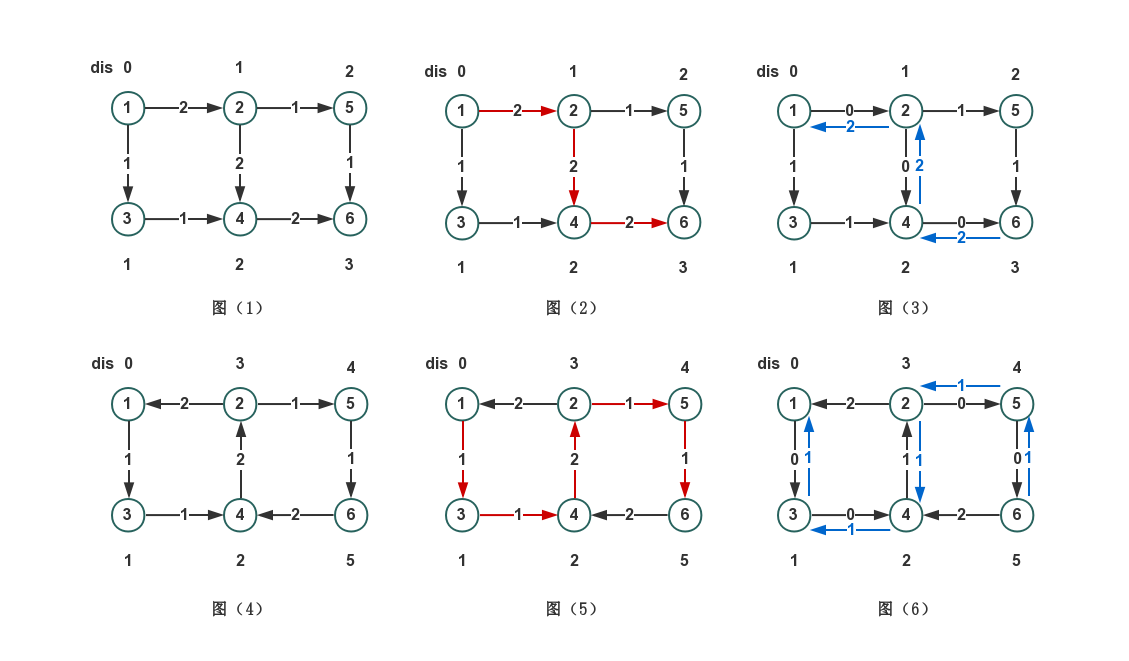# 网络流基础

Wider · 1月27日 · 2020年 · · 4581次已读

# 网络流基础

## 网络流问题

• 源点：有n个点，有m条有向边，有一个点很特殊，只出不进，叫做源点。
• 汇点：另一个点也很特殊，只进不出，叫做汇点。
• 容量和流量：每条有向边上有两个量，容量和流量，从i到j的容量通常用c[i,j]表示,流量则通常是f[i,j]。
• 最大流：通俗点解释，就好比你有很多货物要从源点点运到汇点点，有向图中的一条边代表一条公路，每条公路有固定的货物装载限制（容量），对每条公路你只能运输一定数量的货物，问你每一次运输最多运到汇点点多少货物。

### 网络流的性质

• 容量限制：f[u,v]<=c[u,v]
• 反对称性：f[u,v] = - f[v,u]
• 流量平衡：对于不是源点也不是汇点的任意结点,流入该结点的流量和等于流出该结点的流量和。

### 增广路

• 在 P 的所有前向弧 <u, v> 上, , 即 P+ 中每一条弧都是非饱和弧;
• 在 P 的所有后向弧 <u, v> 上, , 即 P– 中每一条弧是非零流弧。

### 最小割最大流定理

#### 最小割最大流定理

• 任意一个流都小于等于任意一个割
• 构造出一个流等于一个割
• 在一张流量图G中，最大流=最小割。

## 网络流问题解决方法

FF方法(Ford-Fulkerson)

### 基本思想

FF方法的基本流程是 :

• (1) 取一个可行流 f 作为初始流(如果没有给定初始流,则取零流 f= { 0 }作为初始流);
• (2) 寻找关于 f 的增广路 P,如果找到,则沿着这条增广路 P 将 f 改进成一个更大的流, 并建立相应的反向弧;
• (3) 重复第(2)步直到 f 不存在增广路为止。### 算法一：EK算法（EdmondsKarp）

#### 代码

#include<iostream>
#include<cstdio>
#include<cstring>
#include<queue>
#define INF 0x7fffffff
#define N 10010
#define M 100010
using namespace std;
int n,m,ss,tt;
struct Edge{int to;int next;int value;}e[M<<1];
struct Pre{int node;int id;}pre[M<<1];//pre[i].node表示编号为i的点最短路的上一个点,pre[i].id表示最短路上连接i点的边的编号
bool vis[N];
queue<int> q;
{
cnt++;
e[cnt].to=to;
e[cnt].value=value;
}
bool bfs(int s,int t)//用来寻找s,t的最短路并记录,如果s,t不连通则返回0
{
q=queue<int>();//清空队列
memset(vis,0,sizeof(vis));
memset(pre,-1,sizeof(pre));
pre[s].node=s;
vis[s]=1;
q.push(s);
while(!q.empty())
{
int x=q.front();
q.pop();
{
int now=e[i].to;
if(!vis[now]&&e[i].value)//忽略流量为0的边
{
pre[now].node=x;//用pre记录最短路
pre[now].id=i;
vis[now]=1;
if(now==t)return 1;//找到
q.push(now);
}
}
}
return 0;
}

int EK(int s,int t)
{
int ans=0;
while(bfs(s,t))
{
int minv=INF;
for(int i=t;i!=s;i=pre[i].node)
minv=min(minv,e[pre[i].id].value);
for(int i=t;i!=s;i=pre[i].node)
{
e[pre[i].id].value-=minv;
e[pre[i].id^1].value+=minv;//x^1表示x边的反向边,此方法仅在边的编号从0开始时有效
}
ans+=minv;
}
return ans;
}
int main()
{
scanf("%d%d%d%d",&n,&m,&ss,&tt);
for(int i=1;i<=m;i++)
{
int a,b,c;
scanf("%d%d%d",&a,&b,&c);
}
printf("%d\n",EK(ss,tt));
return 0;
}


### 算法二：Dinic算法

#### 算法思路• 图1，bfs计算dis
• 图2，dfs按最短路找到t点，累加路径上的最小容量
• 图3，回溯，顺便更新正边和反向边的边权
• 无其他路径，回溯到源点
• 图4，再次bfs更新dis
• 图5，dfs按最短路找到t点，累加路径上的最小容量
• 图6，回溯，顺便更新正边和反向边的边权
• 无符合要求的其他路径，回溯到源点
• 再次bfs，发现s和t不连通，结束算法

#### 代码

#include<iostream>
#include<cstdio>
#include<cstring>
#include<queue>
#define N 10010
#define M 100010
#define INF 0x7fffffff
using namespace std;
int n,m,ss,tt;
int dis[N];
queue<int> q;

struct Edge{int to;int value;int next;}e[M<<1];
{
cnt++;
e[cnt].to=to;
e[cnt].value=value;
}

bool bfs(int s,int t)//bfs功能和EK算法的相似,不同的是Dinic中的bfs要求出所有点到源点s的最短路dis[i]
{
q=queue<int>();//清空队列
memset(dis,-1,sizeof(dis));
dis[s]=0;
q.push(s);
while(!q.empty())
{
int x=q.front();
q.pop();
{
int now=e[i].to;
if(dis[now]==-1&&e[i].value!=0)
{
dis[now]=dis[x]+1;
q.push(now);
}
}
}
return dis[t]!=-1;
}
int dfs(int x,int t,int maxflow)//表示从x出发寻找到汇点T的增广路，寻找到maxflow流量为止，并相应的增广。返回值为实际增广了多少(因为有可能找不到maxflow流量的增广路)
{
if(x==t)return maxflow;
int ans=0;
{
int now=e[i].to;
if(dis[now]!=dis[x]+1||e[i].value==0||ans>=maxflow)continue;
int f=dfs(now,t,min(e[i].value,maxflow-ans));
e[i].value-=f;
e[i^1].value+=f;
ans+=f;
}
return ans;
}
int Dinic(int s,int t)
{
int ans=0;
while(bfs(s,t))
ans+=dfs(s,t,INF);
return ans;
}
int main()
{
scanf("%d%d%d%d",&n,&m,&ss,&tt);
for(int i=1;i<=m;i++)
{
int a,b,c;
scanf("%d%d%d",&a,&b,&c);
}
printf("%d\n",Dinic(ss,tt));
return 0;
}


#### 优化代码(其他代码不发生变化)

int cur[N];

int dfs(int x,int t,int maxflow)
{
if(x==t)return maxflow;
int ans=0;
for(int i=cur[x];i>-1;i=e[i].next)
{
int now=e[i].to;
if(dis[now]!=dis[x]+1||e[i].value==0||ans>=maxflow)continue;
cur[x]=i;//此路可行，记录此路
int f=dfs(now,t,min(e[i].value,maxflow-ans));
e[i].value-=f;
e[i^1].value+=f;
ans+=f;
}
return ans;
}
int Dinic(int s,int t)
{
int ans=0;
while(bfs(s,t))
{